### Breaker Switching Phenomena and TRV

Here we discuss the phenomena associated with breaker switching. One will be able to better appreciate this article if little knowledge of Arcing in Circuit Breaker is developed first. So before proceeding further it is better to go through the above link. At this point perhaps we are aware that it is important to understand the switching and arc quenching phenomena in a Circuit Breaker. Here is illustrated the main concept of the subject without much attention to the mathematics involved. Of course as usual the aim is to keep the material simple.

Circuit breaker switching results in either breaking the circuit or making the circuit. After a circuit breaker is closed or opened the configuration of the system changes. For example by opening a breaker a part of the network may be de-energised or isolated or a load may be disconnected. The breakers also automatically open when a fault happens in the network. On occurrence of fault the trip signal initiates breaker mechanism so that the breaker contacts separate, and arc is formed between the contacts. The arc is extinguished at current zero. But there are  chances of re-striking, how and why restrike, is the main subject of discussion here. The analysis is not universal. Although one analysis can not be applied to every circuit configuration or situation but still it is enough for developing the main concept of successful arc extinction. Here for illustration we have chosen the case when a fault happens in a line and the line breaker trips to isolate the fault from the source. For illustration we have chosen this case because under faulted condition the breaker is under severe stress which the breaker design should meet to successfully operate. The figure-A below is the circuit with  equivalent elements and fault illustrated.

In the circuit shown, L is the equivalent inductance of the line which may include the transformer reactance up to the breaker. The line resistance being small is first neglected. The impact of line resistance is also important and will be revealed later. C is the equivalent capacitance at the breaker terminal towards source side. The capacitance is mainly due to the equipment bushings. When the arc between the breaker poles is extinguished, another capacitance between the poles exist. It should be realized that due to the dead fault, the capacitance between the poles also contribute to the capacitance C.

Let a fault happens at the load end as shown. The fault is dead short circuit (shown by double headed arrow). The breaker receives trip signal, the tripping mechanism operates and the breaker moving contact starts moving away from the fixed contact. An arc is formed between the contacts. The fault current is fed through the arc as long as arc exist. Being a dead short circuit the fault resistance is assumed zero. So the equivalent circuit of this faulted case is redrawn as in Fig-B(i).

When feeding fault the circuit is purely inductive (ignoring little resistance). The circuit being purely inductive, the current lags the voltage wave V by 90 degrees. The voltage across the poles of the breaker is the voltage drop across the arc. In a high voltage circuit this arc voltage is negligible in comparison to line voltage. So we neglect the voltage drop across the arc. Hence, during arcing the voltage difference between the poles of the circuit breaker is the voltage drop across the arc. Due to dead short circuit voltage across the fault is assumed zero. Hence the voltage across the capacitance is almost same as voltage difference between the poles of the breaker which is zero (Vb = 0). The wave shapes of system voltage, current and voltage across the poles of breaker (violet color) is shown in Fig-C (upto point O).

The arc is extinguished at current zero (point O in Fig-C).  Just at the instant of current zero the arc is extinguished but supply voltage is at its peak. It should be clear that the voltage across the poles of breaker is the voltage across the capacitance C. So just at the point of arc extinction (point O) the voltage across the poles of the breaker is same as the voltage across the capacitance which is zero.

For the new state that is after the point of arc extinction (after point O in fig-C) the circuit is shown in Fig-B(ii) with direction of current shown. In this new configuration the voltage across the capacitance which is the same as the voltage across the breaker terminals tries to approach the system voltage by flow of current through the inductance and capacitance (neglecting the resistance of line). The voltage across the capacitance which is the voltage across the breaker poles approaches the system voltage in an oscillatory manner due to the formation of series LC circuit (See Fig-C). As already said at point 'O' current is zero and arc is extinguished. Before point 'O' the voltage across the poles (same as arc voltage) being negligibly small is shown zero.

The frequency of oscillation of the transient is very high in comparison to the power frequency at 60 Hz or 50 Hz . If fo is the frequency of oscillation of the transient voltage then,

fo= 1 / [2π√(LC)]

ωo= 2πfo

The voltage across the poles of breaker after arc extinction is called as the recovery voltage. But the Transient part just after current zero is called as Transient Recovery Voltage (TRV). After the transient vanishes within few microseconds the voltage across the poles  is called Recovery Voltage which is at power frequency. Look at figure-C how the voltage across the poles (violet) approaches system voltage. It should be noted that the Transient Recovery Voltage is also known as Restriking Voltage.

It is observed that the oscillatory transient is superimposed on power frequency wave shape to give the resultant voltage shape. Due to the low values of both L and C the frequency of oscillation is very high in comparison to power frequency at 60 Hz or 50 Hz. from the figure-C it is shown that the amplitude of oscillation of the transient dies out gradually. Gradual die out of transient is called damping which is due to the presence of the small line/equipment resistance. Due to this small value of resistance we have already considered the circuit as almost purely inductive so fault current lags voltage by 90 degrees.

From the figure it is observed that the voltage increases very rapidly from zero to the first peak. The rate of rise of this Transient Recovery Voltage and the value of the peak voltage are very important for successful arc quenching ability of breaker.

If rate of rise of recovery voltage is higher than the rate of gain in dielectric strength of the medium between the breaker contacts then restrike will happen and arc is again formed between the contacts, feeding the fault for another half cycle at least till next current zero is encountered. Otherwise if rate of gain of dielectric strength is more than rate of rise of recovery voltage than there will be no restrike and no further arc formation. The interrupter should also be able to withstand the peak of Transient Recovery Voltage and Recovery Voltage at power frequency. Otherwise breakdown between the contacts may takes place and arc is again formed. The arc is successfully extinguished if restrike does not happen.

TRV and Recovery voltage depends upon the characteristics and configuration of the circuit.

• As already shown the TRV depends upon the frequency of transient which depends upon L and C. Low L and C means high frequency. And high frequency implies fast rise of TRV.
• The breaker when switching load/elements then the  power factor of the load or element greatly influences the shape of Transient Recovery Voltage and Recovery Voltage.
• The breaker opening may result in independent oscillation of both sides of the breaker. Here the TRV is obtained by subtracting instantaneous voltage of one side from the voltage of other side
• In a three phase system the current in individual phases attain zero values one by one (120 degree phase shift between them) . If the three phase system is ungrounded then the pole that clears first (at current zero) is subjected to a recovery voltage which is 1.5 times the phase voltage.
• The lagging nature of fault current in alternator winding has demagnetising effect. The demagnetising effect results in reduction of emf of alternator. This results in reduction of terminal voltage. Hence the recovery voltage is less than the system voltage.

### Arcing in Circuit Breaker

Electric Arc

For interrupting power supply to a part of a network or circuit, or to clear a fault, circuit breakers are employed. On receipt of trip signal the circuit breaker operating mechanism operates to separate moving contact from the fixed contact (Fig-A). As the moving contact starts moving away from the fixed contact, the contact area of the tips of  both moving contact and fixed contact reduces. But the same current now passes through this reduced contact area. The current density of the contact area increases very much. This situation makes the areas of the tips in contact very hot, may be several thousand degrees celsius. Now as the contacts just separate these hot spots becomes source of electron emission. High energy electrons are emitted from the separated contact tips. This is called thermionic emission.

The other main cause of electron emission is field emission. As the movable contact moves away from the fixed contact voltage difference between the two electrodes (fixed and moving contacts) exist (Fig-B). Which gives rise to an electric field between the electrodes.

Electric Field = V / d

Where  V is the potential difference between the electrodes and d is the separation between the two.

So from the above formula it is clear that just after separation when the movable contact has not moved much away, then d is small so the electric field strength is very high (order of several kV per millimetre). High electric field gives rise to emission of high energy electrons from the contact surface. The high speed electrons emitted bombard the molecule or atoms of the medium and dislodge electrons from the atoms. This is secondary emission.

The high energy electrons so emitted ionise the gas or oil used as medium. Arc plasma is formed between the fixed and moving contacts. The current continues to flow through the arc plasma between the contacts (see Fig-A).

It is clear that just separating the contacts does not automatically break the circuit and flow of current does not stop. The arc is required to be extinguished. More importantly the dielectric strength of the medium between the fixed and moving contacts should be restored quickly, otherwise arc may re-strike between the contacts. This is the job of the arc interruption chamber of the circuit breaker.

Several techniques are employed for the arc extinction. The arc can be extinguished by increasing the resistance of the arc path. This is achieved by lengthening the arc or splitting the arc using one of the techniques and by reducing the diameter of the arc by cooling. By increasing the resistance the voltage drop across the arc increases. It achieves such a value that the supply voltage cannot sustain the voltage drop across the arc and the arc is extinguished.
Also in AC circuit the current varies sinusoidally so the arc is extinguished at next current zero. Although the arc is extinguished but the medium is having enough ionised particles. Hence to stop the re-striking of arc due to Transient Restriking Voltage the space between the separated contacts should rapidly regain dielectric strength. This can be done by blowing gas or air at high speed to the region between the contacts in Gas or Air Blast breaker. In case of oil circuit breaker rapid flow of oil to the contact region helps regain high dielectric strength. Regaining dielectric strength is the result of recombination of ions and electrons.

The working principles of some breakers will be discussed later.

### Circuit Breaker

In last article we discussed common bus schemes used in substations.  We felt the importance of the circuit breaker. Here we will discuss more about the circuit breakers. Although here the primary concern is about the breakers used in HV, EHV or UHV substations the basic is still applicable to low voltage breaker. Also Switchgear is a very common term often used by power system engineers. Switchgear includes circuit breaker, protective devices, and also measuring and control devices.

The main function of the circuit breaker is to break and make the circuit. So theoretically a circuit breaker is a switch. The breaker is rated so that is should be capable to make, carry and break load current during normal operation and interrupt large fault current in abnormal conditions.

Circuit breaker is the main equipment for controlling power flow and safety of other equipments and personnel. The different associated elements and basic functioning of the circuit breaker is illustrated in the simplified Figure-A. The breaker can be operated by a trained substation personnel by pressing the button at the control room. But during the fault condition it trips automatically.

When fault in the line takes place the large fault current is associated with the increase of secondary current in current transformer (CT). This will actuate the relay and relay contact closes. Now as the tripping circuit of the breaker is complete the  trip coil is energised. The energised trip coil initiates breaker mechanism for moving the circuit breaker moving contact away from fixed contact. The Arc formed between the moving contact and fixed contact is extinguished by breaker arc extinguishing mechanism. The breaker is open. From the figure it is clear that the breaker trip circuit can be closed by closing of either of the contacts C1 or C2. While C1 is for manual closing by pressing the button at the control panel, the C2 is closed automatically by breaker relay for abnormal over current condition sensed by CT. These two contacts in parallel fulfil the logic OR function. Applying same reasoning it is easy to think that the breaker can be logically conditioned for tripping on other abnormal conditions.

The trip circuit is supplied with DC battery source. Independent AC source may be used for trip circuit. It should be noted that for high voltage breaker the fault sensing device is outside the circuit breaker where as in case of low voltage breaker the sensing device is accommodated within the breaker enclosure.

During separation of moving contact from fixed contact electric arc is produced between the contacts. Extinguishing of the arc is the most important part of breaker functioning, which greatly influences the breaker design. Actually in low and medium voltage case, the arc extinction is not of much problem. The arc extinguishing is a difficult task in HV and EHV or UHV circuit and is the primary concern for breaker design. The energy stored in the line/circuit inductance is dissipated in the arc and the arc is required to be extinguished reliably.

From the above discussion it is observed that the circuit breaker functioning is comprised of three main components.
• Sensing and tripping circuit
• Operating mechanism
• Arc interruption
The breakers can be classified several ways. The most important classification is the medium used for arc interruption.

For low and medium voltage use, air is mainly used as the medium for arc extinction (In past, oil was also used for 430/220 volt system). In HV, EHV and UHV substations oil and gas is used as arc quenching medium (In the past Air Blast breakers were also often  used for HV/EHV/UHV application). Like low voltage system, Oil Circuit breakers are also becoming obsolete for higher voltage use. Vacuum is also used for arc quenching. Vacuum circuit breakers are usually used for breakers in the range of 3 kV to 38 kV.  In modern EHV and UHV substations  SF6  gas breakers have replaced the Oil circuit breakers and Air Blast circuit breakers. But one can still find Oil Breakers and Air Blast Breakers in many old substations.

SF6 is a superior gas having good dielectric strength and arc quenching ability which has proved to be a better medium for arc quenching in the breaker. An UHV SF6 Circuit Breaker is displayed below.
The circuit breakers are also available as single tank type or separate tank type. In case of separate tank type, each phase has a separate tank. For EHV application separate tank type breakers are preferred.

The circuit breakers can also be classified from the point of view of operating mechanism. The operating mechanism of the circuit breaker may be hydraulic, pneumatic or motor operated types.

The circuit breakers are also classified as live tank type or dead tank type. In case of live tank type breaker the enclosure of the breaker is at line potential. In the dead tank type breaker the enclosure of the breaker is at ground potential. The dead tank type breaker requires additional oil/gas for insulation from the grounded enclosure. Live tank type breaker requires less oil or gas.

More on Circuit Breaker is discussed in subsequent articles.

### Substation Bus Schemes

Introduction:

We already mentioned the different types of substations. Before more in-depth discussion about each type of substation it is better to know few common essential features of a substation. Here we discuss about the bus schemes commonly implemented in an electrical substation. The Bus scheme is the arrangement of overhead bus bar and associated switching equipments in a substation. The operational flexibility and reliability of the substation greatly depends upon the bus scheme.

Here I reiterate that the electric substation is a junction point where usually more than two transmission lines terminate. Actually in most of  EHV and HV substations more than half a dozen of lines terminate. In many large transmission substations the total numbers of lines terminating exceeds one or two dozens. In this scenario obviously the first requirement is avoidance of total shutdown of the substation for the purpose  of maintenance of some equipment(s) or due to fault somewhere. Total shutdown of substation means complete shutdown of all the lines connected to this particular substation. So the switching scheme is adopted depending upon the importance of the substation, reliability requirement, flexibility and future expansion etc.. Of course substation construction and operational cost is also to be considered. Clearly a EHV or UHV transmission substation where large numbers of important lines terminate is extremely important and the substation should be designed to avoid total failure and interruption of minimum numbers of circuits.

There are mainly six bus schemes. these are:
• Single Bus
• Main Bus and Transfer Bus
• Double Bus Double Breaker
• Double Bus Single Breaker
• Ring Bus
• Breaker and Half
Before we proceed further I would like to discuss in brief about the Circuit Breaker and Isolator. It will be helpful for novices. See the figure below where two buses are connected by circuit breakers and isolators as shown. A circuit breaker is a device whose main purpose is to break the circuit carrying load current or fault current. As the breaker is opened then current is interrupted in the circuit. But it is not safe to work with opened breaker as one or both sides of the breaker terminals may be still energised. The breaker is then isolated from the rest of the circuit by opening the isolators on both sides of breaker. The isolators are used to isolate the breaker or circuit.  It should be remembered that the isolators are never opened or closed to interrupt or make the circuit. That means when the circuit is to be made on, first the isolators on both sides of a breaker are closed then breaker is closed to allow current flow. When the circuit is to be made off or interrupted, first the breaker is opened(tripped), hence load current is interrupted. Then to isolate the breaker, isolators are opened. Isolators are designed to interrupt small current. Breakers are designed to interrupt large load current and heavy fault current. Both breaker and isolator carry load current in normal state.
Single Bus

As the name indicate the substation with this configuration has a single bus (Fig-B). All the circuits are connected to this bus.  A fault on the bus or between the bus and a breaker results in the outage of the entire bus or substation. Failure of any breaker also results in outage of the entire bus. Maintenance of any circuit breaker requires shutdown of the corresponding circuit/line and maintenance of bus requires complete shutdown of the bus. A bypass switch across the breaker should be used for maintenance of the corresponding breaker. This case the protection system is disabled.

Single Bus configuration is the simplest and least cost of all configurations. The system can be easily expanded. This configuration requires less area. The reliability of this system being low, it is not to be implemented in the substation where high reliability is expected. Large substations usually do not utilize this scheme.  By sectionalising of the bus the reliability and availability of the single bus system can be improved.

In this scheme one more bus is added. See Figure-C  how the equipments are arranged and circuits are connected between main and transfer bus. In this arrangement one more breaker may be used, known as tie circuit breaker. No circuit is associated with this tie breaker.

When the tie CB is not present, for maintenance of a circuit breaker, the transfer bus is energized by closing the isolator switches to transfer bus. Then the breaker to undergo maintenance is opened and isolated (opening isolators on both sides of CB) for maintenance. In this arrangement there is no protection for the circuits. The circuits can be protected from outside the substation.

When the tie breaker is present, for maintenance of a breaker the transfer bus is energised by closing the tie breaker. Then the isolator near the transfer bus of the breaker of circuit to be maintained is closed. Now the breaker to be maintained is opened. Then corresponding isolators on both sides of breaker are opened. The breaker is removed for maintenance.  The circuit is transferred to transfer bus. Remember that the isolator to the transfer bus corresponding to the breaker not to be maintained remain open. Here the tie breaker protects the circuit in place of removed breaker. In this scheme the relay setting is quite complex due to the requirement of the tie breaker to handle each situation for maintenance of any of the other breakers. This scheme is somewhat more costly than the single bus scheme but is more reliable. The scheme can be easily expanded. The switching procedure is complicated for maintenance of any circuit breaker. Failure of a breaker or fault on the bus results in outage of complete substation.

Double Bus Double Breaker

In this scheme there are two buses and two circuit breakers per circuit are used (See Fig-D). In normal state both the buses are energised. Any circuit breaker can be removed for maintenance without interruption of the corresponding circuit. Also the failure of one of the two buses does not interrupt any circuit as all the circuits can be fed from the remaining bus and isolating the failed bus. By shifting circuit from one bus to other the loading on the buses can be balanced.
The substation with this configuration requires twice as much equipments as single bus scheme. This scheme has high reliability. But due to more equipments this scheme is costly and requires more space. This scheme is usually used at EHV transmission substation or generating station where high reliability is required.

Double Bus Single Breaker

This scheme is shown in Fig-E. This scheme has two buses. Each circuit has one breaker and connected to both buses by isolators as shown. There is one tie breaker between two buses. The tie breaker is normally closed. For the tie breaker in closed position the circuit can be connected to either of the buses by closing the corresponding switch. It is clear that fault on one bus requires isolation of the bus and the circuits are fed from the other bus.

From the figure you can guess that the configuration has some improvement over the single bus system. This arrangement has more flexibility in operation than the single bus scheme. This scheme is costlier and requires more space than the single bus scheme. Many EHV transmission substations use this scheme with an additional transfer bus.

Ring Bus

The Ring Bus configuration is shown in Fig-F. The breakers are so connected and forms a ring. There are isolators on both sides of each breaker. Circuits terminate between the breakers. The number of breakers is same as the numbers of circuits. Each of the circuits in ring bus system is fed from both sides. Any of the breaker can be opened and isolated for maintenance without interrupting any of the circuits. A fault on any of the circuit is isolated by tripping of two breakers on both sides of the circuit. By tripping the two breakers only the faulted circuit is isolated and all other circuits continue to operate in open ring state. This scheme has good operational flexibility and high reliability. The main disadvantage is that when a fault happens and the ring is split and may result into two isolated sections. Each of these two sections may not have the proper combination of source and load circuits. To avoid this as far as possible the source and load circuits should be connected side by side (see figure). The ring bus scheme can be expanded to accommodate more circuits. The ring bus scheme is not suitable for more than 6 circuits (although possible). When expansion of the substation is required to accommodate more circuits, the ring bus scheme can be easily expanded to One and Half Breaker(See below and compare) scheme. The scheme is required to be planned properly to avoid difficulties in future expansion.

Breaker and Half

The Breaker and Half scheme has two main buses (Fig G). Both the buses are normally energised. Three breakers are connected between the buses. The circuits are terminated between the breakers as shown. In this bus configuration for two circuits three numbers of breakers are required. Hence it is called one and half scheme.  It is something like, for controlling one circuit we require one full and a half breakers. The middle breaker is shared by both the circuits. Like the ring bus scheme here also each circuit is fed from both the buses.

Any of the breakers can be opened and removed for maintenance purposes without interrupting supply to any of the circuits. Also one of the two buses can be removed for maintenance without interruption of the service to any of the circuits. If fault happens on a bus it is isolated without interruption of supply to any of the circuits. If the middle circuit breaker fails then the breakers adjacent to the buses are tripped so interrupting both the circuits. But if a breaker adjacent to the bus fails then the tripping of middle breaker does not interrupt power supply to circuit associated with healthy breaker. Only the circuit associated with failed breaker is interrupted.

This configuration is very flexible and highly reliable. The relaying of the scheme is complicated as the middle breaker is associated with both the circuits. This scheme is economical in comparison to Double Bus Double Breaker scheme. This scheme also require more space in comparison to other schemes to accommodate more equipments.

In one substation you can find two or more schemes implemented as per the requirement. In most of the modern substations it is usual to add one transfer bus in most of the schemes above. Which enhances the availability and maintainability of the system and operational flexibility

### Substation Planning and Siting

We have already discussed in brief about different types of electrical substations. Now we will discuss about the main factors influencing selection of substation. The discussion is mainly for transmission substation which requires many factors to be considered before selecting the site, size etc. The smaller or distribution substation has fewer requirements so only some of the points are required to be considered.

A substation is like a junction point in a power system. Many transmission lines at different voltage levels terminate at a substation. Shut down of the substation means shutdown of all the lines terminating at the substation. Clearly a substation is a very important unit of power system.

A transmission substation may be comprised of  one or more of the following main sections depending on its size and importance. These are Switchyards, Control rooms, Office building and Colony for employees. See the figure at the bottom. The substation planning requires several factors to be considered. A substation which is not properly planned or Ill designed may face several problems, in particular if future expansion or renovation of the switchyard is required. Proper design of substation also enables it to operate in normal state for maximum possible time so avoiding overloading to the maximum. It is true that many substations undergo expansion and renovation with time. So for substation planning future expansion must be considered.

There are several factors that need to be considered for deciding for a substation. Some of these requirements are actually the purpose of the substation while the others are the requirements to fulfill the purpose.

Some important primary factors in the design of substation are operational flexibility, supply reliability, secutity and short circuit withstand capability etc.

One important factor to be considered first is the site selection. Substation design and some equipment selection depends on site selection. Hence it has a bigger influence on the cost of substation. The orientation of the lines to be terminated at the substation also decides the substation orientation and equipment arrangement.

The site should be near the load center keeping in view the future load growth.

Some general factors to be considered are listed. It should be remembered that some of the factors are actually interdependent.
• Access road to the site for smooth movement of construction machines, equipments and transformers. Good Roadways to construction site and shorter distance to rail head are desired.
• The site should be chosen to avoid soil filling, earth removal etc. The requirement of soil filling and earth removal takes time and increases total cost of substation
• Historical data of worst flood is taken into account to avoid water logging of the substation in case of possibility of flood. Flood plains and wetlands are avoided.
• Atmospheric conditions like salt and suspended chemical contaminants influence selection of equipments and maintenance requirements.
• Interference with communication signals. The construction company have to take permission from the appropriate authority.
• Electric and magnetic field strength are of particular concern especially for Ultra High Voltage (UHV) systems at 765 kV,1200 kV or above. Research organisations has shown the impact of strong Electric/magnetic fields due to UHV substations and lines on human health. Such new concerns are also required to be addressed properly
• Forest land, sanctuaries and national parks are avoided. Almost all governments has laid stringent rules to comply for approval of forest land and wild life sanctuary. The usual process takes time to get approval from the concerned authorities. This process delays the construction activities.
• Approval is also required from aviation authority. Substation should be away from airport and defence establishments.
• Water supply and sewage system are the two most important facilities to be given due consideration.
Some other factors related to the general public:
• The substation should be located far from the crowded places. Efforts are always made to locate transmission substations outside the city areas.
• The locals should be made aware of the upcoming substation. To avoid public resentment it is better to involve the local people in the process. If required they should be educated and trained. Many times the local people also plays an important role to check vandalism and theft.
• Heritage sites and tourist spots are avoided.
• Electric substation is a source of noise. While charged transformers, reactors and EHV lines are sources of continuous hissing noise, operation of different equipment  also emit sudden noise. The design should be adopted to tackle the issues by complying to the standards set by the appropriate authority for reduction of noise pollution and avoid public resentments.
• Landscaping should be done to keep the substation out of direct view of common people.

Finally it is safety of man and machine. The safety of the personnel involved both in construction phase, operational safety and safety of public is to be followed as per the safety rules and regulations framed by international bodies and local authorities. These rules are not to be compromised. Moreover the manufacturer  instruction manuals,  safety procedures and other documents for equipment and machines are to be referred.

A substation should have adequate arrangement of fire fighting system. It is the ultimate safety measure of any substation. The fire fighting system should be suitable for dealing with fire due to electrical arc. Usually costly equipments like transformer should have Nitrogen gas or high speed water jet as fire fighting system.

In the figure below is a conceptual substation

The substation has 400 kV, 220 kV and 33 kV switchyards. Here some of the equipments/parts are identified. There are two 400/220 kV, 315 MVA Inter Connecting Transformers (ICT). One 220/33 kV, 100 MVA step down transformer is used for feeding the distribution network. The double arrow-head lines represent control and data channels between switchyard equipments and control room.

### Autotransformer

Introduction

Previously we already discussed basics of single phase transformer and three phase transformer. We also discussed about three phase transformer connections or Vector Groups. Now, here we will discuss about auto-transformers. Auto transformers have several applications. But first we will develop some basic concepts of autotransformer. Before proceeding further one should have some basic knowledge of transformer.

Auto transformers can be made in two ways. In one way it can be realized by additively connecting the primary and secondary windings of the two winding transformer. In other way the autotransformers can be thought of built as a single unit with one continuous winding. We will start from first approach and move to second.

Single Phase Autotransformer

Let us first consider a normal single phase two winding transformer. The schematic diagram of a  transformer is shown in Fig-A(i). We will obtain an autotransformer from this usual transformer.

Let the primary side and secondary side voltage ratings are respectively V1, I and V2, I respectively. Number of turns in primary and secondary side are N1 and Nrespectively. Now let us connect the transformers as shown in Fig-A(ii) with additive polarity. For the analysis purpose for better visibility we rearrange the windings in Fig-A(ii) as shown in Fig-B. Of course here we have shown a load connected across secondary

For the purpose of simplifying the analysis the transformer is considered as ideal. (In case of power transformer the ideal transformer analysis gives quite accurate result). The autotransformer analysis can be very simple if you recall two important concepts of a transformer.

• The voltage developed in the windings are dependent on the flux linkages. The windings are wound on the same magnetic core so they link the same flux. Hence
V/ N1= V/ N2

So whenever voltage V1 exist across primary winding, then voltage V2 will be induced across the
secondary winding irrespective of changes in connections.

•  Similarly the magnetic circuit demands that mmf should be balanced. It implies the primary side ampere turn should equal the secondary side ampere turn. Hence
I. N1= I. N2

It means current  I2 that flows  in secondary winding is associated with current  Iin primary winding according to above mmf balance formula.

In Fig-B the primary  of autotransformer is taken across both the windings where as secondary is across N2 winding.
The autotransformer is so loaded that the secondary current is I1+I2 . It makes the current flowing in the windings as I1 and I2   which are the rated values.
using KCL and KVL if the primary side voltage and current of the auto transformer is Vp and Ip and secondary side voltage and current of the auto transformer is Vs and Is then,

V= V1+V
Ip = I
Vs = V
Is = I1+I2.

Now the capacity of the autotransformer is (V1+V2).I1 or (I1+I2).V2

Using the voltage ratio and mmf balance formula it is quite easy to show that the autotransformer capacity formula can be simplified as

The capacity of autotransformer = Sa = (V+ V2).I= (I+ I2).V2 = V1 I1 (1 + N/ N1)
= V2 I2 (1 + N/ N1)

Where as the capacity of our original transformer = S =V1 I1 = V2 I2

So,   Sa = S(1 + N/ N1)

But (1 + N2 /N1) is always greater than 1.
Hence by forming an autotransformer the capacity of the resulting autotransformer is always more than the original isolated winding type transformer. In the so formed autotransformer it should be remembered that the voltage and current through the windings remains as before i.e the rated values. From the above formula it is clear that larger value of N2/N1  gives larger capacity of the autotransformer.

In this case the voltage ratio of autotransformer =  (V1+V2)/ V2= V1 / V +1 = (N1 / N) + 1

Hence   V/ Vs =  (N1 / N) +1 =   (N1 / N) +1

From this formula it is clear that if  N2 /N1 is made large to increase the capacity then the voltage ratio between primary and secondary of autotransformer approaches 1. For this reason auto transformers are advantageous for use in power network when the voltage ratio between both sides is near unity. It is used in grid substations as interconnecting transformers (ICT). The autotransformers are used to interconnect two  different voltage levels. For example interconnection of 400kV and 220kV, 735kV and 345kV and 765kV and 400kV etc.. The voltage ratio should be less than 3:1 for more advantageous use.

Again look at the formula,
I. N1= I. N2

As we said  N2 /N1 should be large for adavantageous use.

To achieve it, N2  should be much greater than N1

It implies that  I2   will be much less than I1

The current I2  flows in the common winding of the autotransformer. Hence the auto transformer can be designed with N2 number of turns made of conductor of smaller cross section area, so resulting in a big saving.The autotransformers are cheaper and lighter in comparison to two winding transformers.

Just looking at the sketch you may think that instead of connecting two windings in additive ways. Why should not it be made of single winding and one terminal brought out from the middle as per requirement. Yes this is true and the autotransformer can be thought of made of a single winding having a part of winding common to primary and secondary .

Sometimes this method is used to obtain a variable secondary voltage. This case it is so designed that the middle contact can smoothly slides over the coil. It is commonly used in the academic electrical laboratories. This is usually called as Variac(Fig-C) or Dimmerstat. There are some other terminologies adopted by different manufacturers.  In this design it is not possible to adopt conductors of two different cross sectional area as in case of ICT where turns ratio is fixed(due to fixed voltage ratio) between primary and secondary.

Autotransformers are also used for voltage regulation in distribution networks, for starting of induction motors and as lighting dimmers. Autotransformers are also used in electric traction.

One main disadvantage about autotransformer is that the primary and secondary are electrically connected.  So the electrical disturbance i.e high voltage transients from one side can be easily transmitted to the other side.
The other disadvantage is that the impedance of the autotransformer is considerably low, so the short circuit current will be more. More over an open circuit in common winding results in full primary side voltage across the load which is harmful.

Three Phase Autotransformer

First thing is that the theory of single phase autotransformer is the basis of three phase autotransformer. Three single phase autotransfor bank can be used for forming a three phase transformer or a single unit  three phase autotransformer can be built. The three phase autotransformers (see Fig-D) are connected in star-star(Wye-Wye). If the autotransformers are connected as Delta-Delta, then phase difference between primary and secondary exist which is not desired (See Vector Groups).

In three phase Y-Y connected power autotransformers an additional delta connected winding is used to take care of zero sequence currents (for unbalanced systems), and third harmonic currents.

Although we discussed here is for one particular case still we revealed the general approach. If you wish to connect load across the other winding, then you can proceed in a similar way.

Moreover the above analysis is for step down case. You can easily analyze for step up case by interchanging the position of source and load. In this case the direction of I1+I2 is reversed so also the directions of Iand I2. It should be recalled again that the change of direction of current in series winding is associated with change of direction of current in common winding to satisfy  mmf balance.

### Complex Power and Power Triangle

Introduction

In the last article we discussed the basics of Electrical Power and related terminologies. Here we will develop the concept of  Complex Power and Power Triangle. These two are very important concepts used frequently by power engineers. As this article requires the knowledge of previous article so it is advised to at least have a look  at the last article.

From the previous article the following points are clear.
• The instantaneous power p is composed of real power and reactive power.
• The time average value of instantaneous power is the real power (true power) is |V | | I | cos φ
• The instantaneous reactive power oscillates about the horizontal axis, so its average value is zero
• The maximum value of the reactive power is |V | | I | sin φ

It should be remembered that real power is the average value and the reactive power is maximum value.

Complex Power

In power system analysis the concept of Complex Power is frequently used to calculate the real and reactive power.

This is a very simple and important representation of real and reactive power when voltage and current phasors are known. Complex Power is defined as the product of Voltage phasor and conjugate of current phasor. See Fig-A

Let voltage across a load is represented by phasor V  and  current through the load is I.

If S is the complex power then,

S = V . I*

V is the phasor representation of voltage and I* is the conjugate of current phasor.

So if  V is the reference phasor then V can be written as |V| ∠0.
(Usually one phasor is taken reference which makes zero degrees with real axis. It eliminates the necessity of introducing a non zero phase angle for voltage)

Let current lags voltage by an angle φ, so  I = | I | ∠-φ
(current phasor makes -φ degrees with real axis)

I*=  | I | ∠φ
So,
S = |V|  | I | ∠(0+φ) =  |V|  | I | ∠φ

(For multiplication of phasors we have considered polar form to facilitate calculation)

Writting the above formula for S in rectangular form we get

S =  |V|  | I | cos φ  +  j  |V|  | I | sin φ

The real part of complex power S is |V| | I | cos φ which is the real power or average power and the imaginary part  |V| | I | sin φ is the reactive power.

So,              S = P + j Q

Where        P = |V| | I | cos φ    and    Q = |V| | I | sin φ

It should be noted that S is considered here as a complex number. The real part P is average power which is the average value, where as imaginary part is reactive power which is a maximum value. So I do not want to discuss further and call S as phasor. If you like more trouble I also advise you to read my article about phasor  or some other articles on phasor and complex numbers.

Returning to the main point, from the above formula it is sure that P is always more than zero. Q is positive when φ is positive or current lags voltage by φ degrees. This is the case of inductive load. We previously said that inductance and capacitance do not consume power. The power system engineers often say about reactive power consumption and generation. It is said that inductive loads consume reactive power and capacitors produce reactive power. This incorrect terminology creates confusion.

The fact is that most of the loads are inductive and they unnecessarily draw more current from source. Although in each cycle both inductance and capacitance draw power from the source and return same amount of power to the source but the behavior of inductance and capacitance are opposing to each other. When capacitors are connected in parallel to inductive load the power requirement of inductive load is supplied by capacitor in half cycle and in next half cycle the reverse happens. Depending upon the values of capacitor this power requirement of inductance in the load may be fully or partially satisfied. If partially satisfied the rest will be drawn from the distant source. By properly selecting the capacitance the maximum value of reactive power (Q) drawn from the distant source (or returned to the distant source) is reduced. This reduction in reactive power results in reduction of line current so the reduction of losses in transmission line and improvement in voltage at load end.

Power Triangle

Returning to the complex power formula, P, Q and S are represented in a power triangle as shown in figure below.

S is the hypotenuse of the triangle, known as Apparent Power. The value of apparent power is |V|| I |

or    |S| = |V|| I |

It is measured in VoltAmp or VA.

P is measured in watt and Q is measured in VoltAmp-Reactive or VAR. In power systems instead of these smaller units larger units like Megawatt, MVAR and MVA is used.

The ratio of real power and apparent power is the power factor of the load.

power factor = Cos φ = |P| / |S|

= |P| / √(P 2+Q 2)

The reactive power Q and apparent power S are also important in power system analysis. As just shown above the control of reactive power is important to maintain the voltage within the allowed limits. Apparent power is important for rating the electrical equipment or machines.

Total Power of Parallel Circuits

In real world the loads are usually connected in parallel. Here we will show the total power consumed by parallel branches. See Figure-C. It has two branches.

First we have to draw the individual power triangles for each branch. Next the power triangles are arranged back to back keeping real power in positive x direction as shown. The total power consumed is obtained by connecting starting point O to the tip of last triangle. This is actually the result of addition of complex numbers.

If   S= P+j Q1
S= P+j Q2

Then,  S = S1+  S

or    S = (P1+  P ) + j  (Q1+  Q)

P = P1+  P
Q = Q1+  Q

In the above diagram S1  P1 ,   Q1 and φ 1 correspond to branch1 and S P2 ,   Q2 and φ  correspond to branch2.
S, P, Q and φ correspond to total power consumption as seen by the generator

### Instantaneous, Average, Real and Reactive Power

Forward

We discussed and developed some important concepts of transmission lines in last few articles. Last time we discussed about long transmission lines. Here we discuss a simple but important basic concept Electric Power. This will refresh our knowledge before we move further.

Electric Power has same meaning as mechanical power but here the power or energy that we are concerned is in Electrical form. We often encounter terms like instantaneous, average, total, real, reactive, apparent and complex power or simply power. What they mean? how are they related ? That we will discuss here and in next article.

DC Circuit

As long as our analysis is restricted to Direct Current(DC) circuit the power consumed by the resistance load is the product of voltage across the resistance and current flowing through the resistance. It is really simple.

P = V . I
The power consumed by the load is the product of voltage across the load and current drawn by the load (Fig-A). Or the Power supplied by the DC source (battery/cell) is the product of voltage across the cell and current supplied by the cell. Both are equal in our example figure(considering ideal battery of zero internal resistance). The law of energy conservation implies power supplied by the source must be same as power consumed by the circuit. In DC circuit case instantaneous power is same as average power.
AC Circuit

In AC circuit analysis, what is this power that we talk about. The main problem is that the AC voltage and current varies sinusoidally with time. Moreover the presence of circuit reactive elements like Inductor and capacitor shift the current wave with respect to voltage wave (angle of phase difference).

Power is rate at which energy is consumed by load or produced by generator. Whether it is DC circuit or AC circuit, the value of instantaneous power is obtained by multiplying instantaneous voltage with instantaneous current. If at any instant of time t the voltage and current values are represented by sine functions as

v = Vm  sin ωt

i = Im  sin (ωt-φ)

Vm and Im  are the maximum values of the sinusoidal voltage and current. Here ω=2 π f
f is the frequency and ω is the angular frequency of rotating voltage or current phasors. It should be clear that for a power system f is usually 50 or 60 Hz
φ is the phase difference between the voltage and current.

As we said the instantaneous power is the product of instantaneous voltage and current, if we name instantaneous power as p then

p = v.i =  Vm  sin ωt  .  Im  sin (ωt-φ)
or  p = Vm Im  sin ωt  sin (ωt-φ)

Applying trigonometric formula 2.sin A.sin B = cos(A-B) - cos (A+B)  we get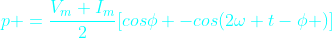It can be written as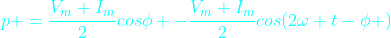This is the equation of instantaneous power

In the Fig-C is drawn all the three waves corresponding to v, i and p. Graphically also we can get the value of instantaneous power (p) at any instant of time t by simply multiplying the value of current i and voltage v at that particular instant t. (You can verify that in the diagram p is negative when either v or i is negative otherwise p is positive. See the points where p is zero). In the graph we have shown horizontal axis as angle φ instead of time t for easy visualization. It should be clear that both way it is correct.

Clearly the instantaneous power p is composed of two terms. The first term is constant because for a given load the phase angle φ is fixed. It does not change unless the load is changed.  The second term  is varying with time sinusoidally due to the presence of the term cos (2ωt-φ). Look that the instantaneous power frequency is twice the frequency of voltage or current.

So the instantaneous power in a single phase circuit varies sinusoidally.

The instantaneous power,  p = constant term + sinusoidal oscillating term.

In one complete period the average of oscillating term is zero.

Then what is the average power within a given time, say one Time Period of the wave?

It is the constant term.

Here is another way to think about the average power.
Just observe that the instantaneous power is negative for a small time. For any time interval you just find the total +ve area A+ (above horizontal-axis (blue line) and below p curve) and total -ve area A- (below horizontal axis and  above p curve). The net area is obtained by subtracting A- from A+. By dividing this net area ( by the time interval T we get the average power(P). You can do this using calculus. What you will ultimately get is only the first term in the above formula for instantaneous power p.

In still another way it is easier to realize that the formula for instantaneous power p has a constant term  (Vm.Im / 2) cos φ and the other sinusoidal term (Vm.Im / 2) cos (2 wt - φ). Actually p is the oscillating power which oscillates about the average constant term  (Vm.Im / 2) cos φ .

So the average power is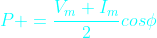The above formula can be written as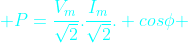Or,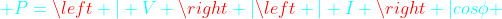here,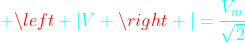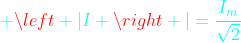V and I are the phasor representation of RMS values* of voltage and current sinusoids. The symbols |V| and |I| are  the magnitudes of phasors V and I. (See at the buttom for definition of RMS value).

This above formula is your favorite formula for useful power that we are most concerned about. This average power formula is used to find the power consumed by the load. The monthly electric energy bill at home is based on this power. The engineers and technicians in power or electrical industry simply use the term power instead of average power. So whenever we simply call power it means average power.

Of course the instantaneous power is oscillating in nature. As we already said it does not oscillates about the horizontal-axis rather about the average power P (cyan color horizontal line).

P will be zero when cos φ =0 or  φ  = 90 degree, that is when the phase angle between voltage and current waves is 90 degrees. It is only when the load is pure inductive or capacitive. In this case the second term only remains in the instantaneous power formula.

From the above figure for some time the power becomes negative that means the load supply energy to source for this period. This is due to the presence of reactive element in load.

The above formula for instantaneous power can be written in another form. This form actually is an attempt to distinguish the oscillating reactive power from the instantaneous power formula.   Rearranging the terms in equation for instantaneous power above we get

p = |V| | I | cos φ (1-cow2ωt) - |V| | I | sin φ sin2ωt

In this equation the first term |V| | I | cos φ (1-cow2ωt) is oscillatory whose average value is |V| | I | cos φ. We already talked about this average power.

The second term |V| | I | sin φ sin2ωt which is also oscillatory but with zero average value. The maximum value of this term is |V| | I | sin φ. This is the so called Reactive power. So Reactive power is the maximum value of a oscillatory power that is repeatedly drawn from the source and again returned to the source within each cycle. So the average of this reactive power is zero.

The average power P is called as Real Power. It is also sometimes called active power.

Real power = P = |V| | I | cos φ

It is usually written as P = VI cos φ. But it should be remembered that V and I are the rms values of voltage and current. For example when we say single phase 220 volt AC it means the rms value of voltage is 220 volts ( it is not maximum value of voltage sinusoid)

Reactive power = Q = |V| | I | sin φ

Real power is measured in Watt and the reactive power is measured in VAR (VoltAmpereReactive). In power sector these units are too small so real power is measured in Megawatt (MW) and reactive power in Megavar (MVAR). The letter R at the end denotes reactive power.

Many times students and practicing engineers are confused about the average power (often simply called power). They think that what they get by multiplying RMS voltage and RMS current is RMS power. No that is wrong. There is no RMS power. RMS power has no meaning or not defined. (Also see definition of RMS value, below at the end). It is average power or real power or true power.

Power In Three phase Balanced System

Let us consider a three phase balanced system. A three phase balanced system is analysed considering only one phase and neutral return. This is called per phase analysis. So the above analysis for single phase is true for balanced three phase case. Let the total power here is Pt. Then we get total three phase power as thrice of single phase case.

P= 3 |V| | I | cos φ

It should be remembered that |V| and | I |  are the per phase values. and φ is the phase angle of load in per phase analysis.

The above formula for balanced three phase system can be written as

P= √3 |Vl| | Il | cos φ

In the above formula Vl and  Il are line voltage and current (Fig-D). This equation is independent of type of three phase load connection i.e delta or star connected load. You have to know the line voltage, line current and phase angle φ as above. This form is very convenient and used often in power calculation.

There is one main difference between the single phase and total three phase power. The instantaneous single phase power is pulsating. In the balanced three phase case, each phase instantaneous power is pulsating but the three pulsating power waves are 120 degrees displaced from each other. At any instant of time the total of these three instantaneous power waves is a constant which is 3 |V| | I | cos φ. So the total power consumed in three phase balanced system is not pulsating. Non-pulsating power also imply the desired non-pulsating torque in case of three phase rotating machines. In large 3-phase motors this is really desired.

*RMS value of AC Sinusoids

The value of AC voltage or current that produces the same heating (or same energy) that is produced if DC voltage or current numerically equal to RMS value of AC is applied instead of AC. This concept helps make the formula for power similar for both DC and AC circuits.

You should read the next article about Power Triangle and Complex Power

### Long Transmission Line

In the last article we discussed short and medium length lines. Now is the time for long transmission lines. For long transmission lines if we apply the formula for medium length line we will get large error. The error becomes larger with longer transmission lines and the method is useless.  So for long lines it becomes important to represent accurately. Hence the long transmission lines are represented by distributed parameters.

Long Line Model

For medium length line we lumped the line impedance at one place. This line impedance which is actually distributed throughout the length of line will be represented here as distributed.  The capacitive current between the line conductors flows throughout the length of line. Which necessitates for distributing the line to line capacitance throughout the length of the line.  But for a balanced three phase system we  analyse per phase analysis. Which necessitates for consideration of phase to neutral capacitance, Both line inductance and capacitance for several configurations are illustrated in previous articles. The equivalent long line representation is shown in Fig-A.

In the diagram the distributed capacitance also automatically requires that the line impedance also distributed. It should be clear from the above figure that in general throughout the length of the line the voltage and current values may vary.

What is the voltage and current at a distance x from the receiving end. See Fig-B Let the voltage and current at a distance x from receiving end are V(x) and I(x) respectively. It should be noted that V(x) and I(x) are phasors.

Let  Z= √(Z/Y)
and   γ =√(ZY)

As Z and Y are complex numbers so in general Z and γ are complex numbers. Z is called the characteristics impedance and γ is the propagation constant. Z is commonly called surge impedance in power sector.

Formula for calculation of Z and Y are already discussed in previous articles.
Z is the series impedance per unit length ( impedance per kilometer or meter)
Y is the shunt Admittance per unit length

Using differential calculus and solving the resulting differential equations it can be shown that,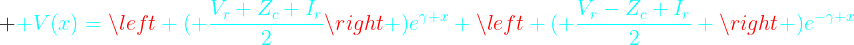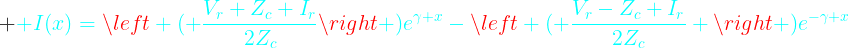The above formula can be rearranged and written in several ways. Rearranging the terms and using the formulas of complex hyperbolic, the above formulas for V(x) and I(x) can be written in the form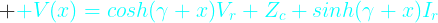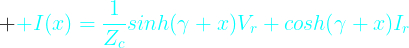To find the voltage Vs and current Is at the sending end, we just have to put l (length of line) in place of x  in the above formulas. So at the sending end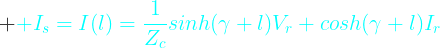If you compare the above equations relating sending and receiving end voltage and current with the corresponding equations discussed in last article for medium length lines then we can easily find ABCD parameters. These are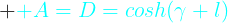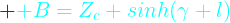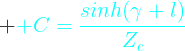You can argue that the long transmission line can also be represented by a nominal  ∏ circuit. Yes a long line can be represented by an equivalent ∏ circuit. It should be remembered that in case of medium length line it is called Nominal ∏. Here it is called equivalent  ∏. This equivalent ∏ is only a convient representation of the actual long line analysis. But Nominal ∏ is an approximation for medium lines. For load flow study and other system studies this equivalent representation is very helpful without sacrificing any accuracy. In Fig-C is shown the equivalent ∏ representation of any line of length l according to long line theory.

It is left as an exercise for you to find Z' and Y' by comparing with Medium length line.

In all the above formulas we used two parameters Zand  γ. These two parameters are very important. Of course these two parameters are derived from our transmission line parameters Z and Y. The characteristics of the long line depends upon these two  parameters.

As already said Zc  and γ  are complex numbers.

Let us consider a case when the load impedance is just equal to the characteristics impedance Zc   then, the receiving end voltage  Vr  =IZc

substituting in above equations we get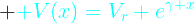and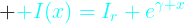let, γ  = α+j β

Dividing V(x) by I(x) we get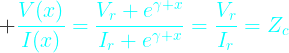From the above equation it is easy to interpret that the impedance as seen at any point of the line is the same as the load impedance that is Z, the characteristics impedance. Moreover from the above equations of  V(x) and I(x) it is clear that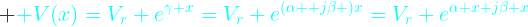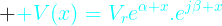The magnitude of voltage is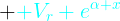Clearly the magnitude of the voltage increases with x. But our x increases from receiving end to sending end. So the voltage increases exponentially from receiving end to sending end.

At the sending end the voltage is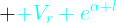The other term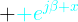is the phasor and only provides phase shift between the voltages at receiving end and at a point x distance from the receiving end. Similar argument can be made for equation of current I(x).

In the above formula of Zc and γ  we put Z(series impedance) and Y(shunt admittance). (recall that Z and Y are the values per unit line length)

Loss Less Line

What will happen for a loss less line. For loss less line R=0 and G=0.
So our above equations reduces to

Observe that for loss less line, Zc becomes a pure resistance. We also know that γ  = α+j β,  for this loss less situation we get

Now our previous voltage and current equations for surge impedance loading reduces to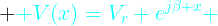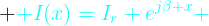As in case of lossy line here also at any distance x from receiving end the ratio of voltage and current is always same that is Zc, the surge impedance of the line. Using the complex algebra you are sure that the magnitude of V(x) is Vr and I(x) is Ir. Which means that for loss less line the voltage and current at any distance x from the receiving end is same. It also implies voltage at sending end is same as voltage at receiving end which is same as voltage at any intermediate point. So Vs = V(x) = Vr. At Surge Impedance Loading the reactive power generated by the line capacitance is equal to the reactive power absorbed by the line inductance for every unit length of line. In Power industry it is said that the voltage profile is flat. So we conclude that for a lossless line the voltage magnitude is same throughout the length of line. As in case of lossy line the term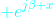is the phasor responsible for phase shift. It is simply giving phase shift to the voltage wave along the length of line. The phase angle between sending and receiving end voltage is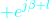. It is clear that if the distance between the sending and receiving end is more then the phase difference between the voltage phasors at both the ends of the line will be more.

In previous articles we already discussed that in case of transmission line how and when we can ignore the line resistance R and  leakage  conductance G. At least for rough estimate of the load carrying capability of transmission line we can presume it lossless. The surge impedance loading is the ideal loading of the line, which is desired keeping in view of the optimised (flat for lossless ideal case) voltage profile of the line.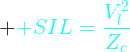Where

It should be recalled that Zc is pure resistance for lossless line.

Vr and Vl are the receiving end phase and line voltage respectively.

Approximate SIL for few nominal voltages
• 132/138 kV  -    50 MW
• 230 kV         -  150 MW
• 345 kV         -  400 MW
• 400 kV         -  500 MW
• 500 kV         -  900 MW
• 765 kV         -  2090 MW

Above values of SIL is true for both 50 Hz and 60 Hz systems.

System planners usually use line loadabilty curve for deciding loading capability of the line. See Fig-D. The relationship between SIL and length in km shown in Fig-D is almost same for all voltage levels.

What is the capacity of the transmission line or how much power it can carry. The power that a transmission line can carry are based on three factors. These are
• Thermal Limit
• Voltage Drop Limit
• Stability Limit
Due to the current flow heat is generated in the line and the line length changes which gives rise to more sag.  Sometimes heating of the line is enough that, later cooling of the line due to less load or environment factors does not make the line regain its actual length. The sagging become permanent. Due to this the minimum clearance of the line to ground decreases which may violates the standard set by the local authority. Also if the load is very high the conductor may be damaged due to excessive heat. All transmission lines has thermal limits. But the thing is that only short lines can approach this limit. Voltage drop and stability limits situation usually do not arise here due to short length. Lines less than 80 km length falls in this category.

For medium length line the loading is mainly limited by allowable voltage drop (usually between 5 to 10 % as set in grid standard). For medium length line the steady state stability limit situation usually does not arises due to lesser length(discussed below). But the length is enough so that the medium length line can encounter the voltage drop limit before reaching thermal limit. By reactive compensation the voltage drop limit can be increased. Lines exceeding 80 km and less than 250 km long belong to this category.

In both lossy and lossless lines it is clear that phase difference between sending and receiving end voltage arises. This angle is called power angle. The power that flows from sending to receiving end depends upon this angle and the magnitude of Vs and Vr. (The magnitude of a phasor V is represented as |V| )

The simplified formula for this power (P) transmitted is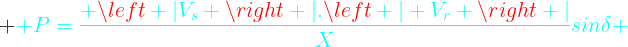X is the equivalent series reactance(ignoring resistance) of the line.

To increase the power to be transmitted, this power angle δ may increase up to 90 degrees. Increasing slightly further, the line becomes unstable and lose synchronism. It is a good practice to operate the lines with sending and receiving ends phase differnce angle (power angle) less than 30 degrees.  Doing so, if in emergency load generation disbalance in adjacent areas occurse this line can take more load by increasing this power angle, so avoiding instability. So operating below 30 degrees we keep more than 60 degree angle margin. From the above formula you can say that if X is made smaller and smaller for any fixed small δ ( small sin δ  imply small δ)  then more power can be transmitted. Hence it is clear that small line reactance X is desired. This small reactance which cannot be made arbitrarily small by line design, definitely limit the power transmission in line.  This loading limit for transmission line is quite less than thermal limit.  So the long lines cannot approach thermal limit, before that other limits come to action. This small power angle corresponds to quite lesser load carrying capacity in comparison to thermal limit. Effort is made by power companies to push the limit towards thermal limit by employing reactive compensation so reducing effective series X further.